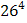### Sample Problem

How many four letter “words” contain a repeated letter?

#### Solution

We could do this by actually calculating all the different ways to have repeated letters. However, this would be an extremely difficult task. Let’s make it easier. Let us instead find the number of four letter “words” and subtract the number of four letter “words” that do not contain a repeated letter. The number of four letter “words” is= 456976. The number of four letter “words” that do not contain repeated letters is 26 × 25 × 24 × 23 = 358800. Thus, the number of four letter “words that contain a repeated letter is 456976 – 358800 = 98176. Good luck if you still want to stick with the normal way.